## Law of Cosines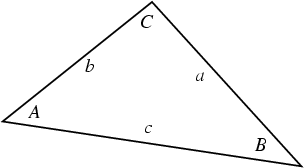Let,, and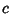be the lengths of the legs of a Triangle opposite Angles,, and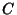. Then the law of cosines states(1)

This law can be derived in a number of ways. The definition of the Dot Product incorporates the law of cosines, so that the length of the Vector fromtois given by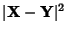(2)(3)(4)

whereis the Angle betweenand.The formula can also be derived using a little geometry and simple algebra. From the above diagram,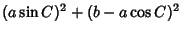(5)

The law of cosines for the sides of a Spherical Triangle states that(6)(7)(8)

(Beyer 1987). The law of cosines for the angles of a Spherical Triangle states that(9)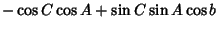(10)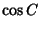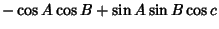(11)

(Beyer 1987).